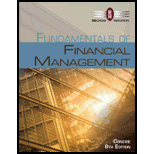# DEFAULT RISK PREMIUM The real risk-f nee rate, r*, is 2.5%. Inflation is expected to average 2.8% a year for the next 4 years, after which time inflation is expected to average 3.75% a year. Assume that there is no maturity risk premium. An 8-year corporate bond has a yield of 8.3%, which includes a liquidity premium of 0.75%. What is its default risk premium?### Fundamentals of Financial Manageme...

8th Edition
Eugene F. Brigham + 1 other
Publisher: Cengage Learning
ISBN: 9781285065137

#### Solutions

Chapter
Section### Fundamentals of Financial Manageme...

8th Edition
Eugene F. Brigham + 1 other
Publisher: Cengage Learning
ISBN: 9781285065137
Chapter 6, Problem 13P
Textbook Problem
5 views

## DEFAULT RISK PREMIUM The real risk-f nee rate, r*, is 2.5%. Inflation is expected to average 2.8% a year for the next 4 years, after which time inflation is expected to average 3.75% a year. Assume that there is no maturity risk premium. An 8-year corporate bond has a yield of 8.3%, which includes a liquidity premium of 0.75%. What is its default risk premium?

Summary Introduction

To identify: The default risk premium.

Default Risk Premium: A premium, which is paid by the borrower to its lender in the form of compensation of lender’s money in the regards of default risk is known as default risk premium.

### Explanation of Solution

Solution:

The items required for the calculation of default risk are yield, real risk-free rate, maturity risk premium and liquidity premium.

The real risk-free rate is 2.5%. (Given)

Yield is 8.3%. (Given)

The liquidity premium is 0.75%. (Given)

The inflation premium is 3.275%. (Working note)

Formula to calculate the default risk premium derives from the formula of actual yield,

r=r*+IP+MRP+DRP+LPDRP=r(r*+IP+MRP+LP)

Where,

• r is the interest rate of treasury bills.
• r* is the real risk-free return.
• MRP is maturity risk premium.
• DRP is default risk premium.

Substitute 8.3% for r, 2.5% for r*, 3.275% for IP, and 0.75% for LP.

DRP=8.3%(2.5%+3.275%+0.75%)=8.3%6.525%=1.775%

The yield on 8 year corporate bond is 1

### Still sussing out bartleby?

Check out a sample textbook solution.

See a sample solution

#### The Solution to Your Study Problems

Bartleby provides explanations to thousands of textbook problems written by our experts, many with advanced degrees!

Get Started

Find more solutions based on key concepts
Differentiate between ROE and ROIC.

Fundamentals of Financial Management, Concise Edition (MindTap Course List)

What are the through-the-computer techniques?

Accounting Information Systems

Differentiate between FOB origin and FOB destination pricing.

Foundations of Business (MindTap Course List)

Are all intangible assets amortized? not, which ones are not? Why?

Intermediate Accounting: Reporting And Analysis

Income taxes are a unique expense of the corporate form of business.

College Accounting, Chapters 1-27 (New in Accounting from Heintz and Parry)